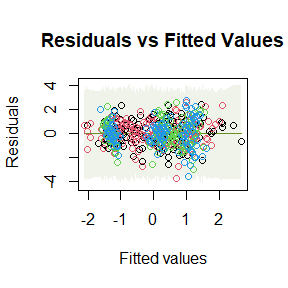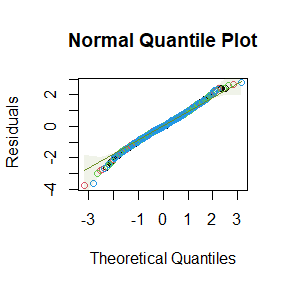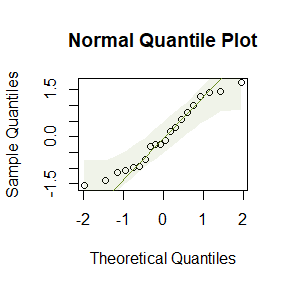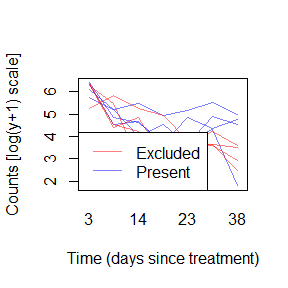Eco-Stats - Code and Data Accompanying the Eco-Stats Text

2020-11-06

The Eco-Stats package contains functions and data supporting the Eco-Stats text (Warton, in press, Springer), and eventually, solutions to exercises. Functions include tools for using simulation envelopes in diagnostic plots, and a function for diagnostic plots of multivariate linear models. Datasets mentioned in the package are included here (where not available elsewhere) and vignette solutions to textbook exercises will be forthcoming at a later time.

Simulation envelopes in plots

The command plotenvelope will standard residual plots for most model objects, but will add global envelopes around points (for quantile plots) and around smoothers (for residual plots) constructed via simulation. These are constructed using the GET package as global envelopes, that is, when model assumptions are correct, 95% of quantile plot envelopes (at confidence level 95%) should contain all datapoints.

library(ecostats)
data(iris)
Y = with(iris, cbind(Sepal.Length,Sepal.Width,Petal.Length,Petal.Width))
iris.mlm=lm(Y~Species,data=iris)
# check normality assumption:
plotenvelope(iris.mlm,n.sim=199)For mlm objects, this function will compute conditional residuals and fitted values, that is, they are computed for each response conditional on all other responses being observed, via the cpredict and cresiduals functions. This is done because the full conditionals of a distribution are known to be diagnostic of joint distributions, hence any violation of the multivariate normality assumption will be expressed as a violation of assumptions of these full conditional models. The full conditionals are well-known to follow a linear model, as a function of all other responses as well as predictors.

The qqenvelope function can be applied for a normal quantile plot, with global envelope, to either a fitted model or a sample of data:

y=rnorm(20)
qqenvelope(y)Datasets

All datasets used in the Eco-Stats text, where not available elsewhere, are supplied here:

data(aphids)
cols=c(rgb(1,0,0,alpha=0.5),rgb(0,0,1,alpha=0.5)) #transparent colours
with(aphids\$oat, interaction.plot(Time,Plot,logcount,legend=FALSE,
col=cols[Treatment], lty=1, ylab="Counts [log(y+1) scale]",
xlab="Time (days since treatment)") )
legend("bottomleft",c("Excluded","Present"),col=cols,lty=1)